﻿ SimMechanics and Forward and Inverse Problem of Dynamics

### SimMechanics and Forward and Inverse Problem of Dynamics

Darina Hroncová, Róbert Rákay, Tomáš Lipták

Journal of Automation and Control

## SimMechanics and Forward and Inverse Problem of Dynamics

Darina Hroncová1,, Róbert Rákay2, Tomáš Lipták1

1Department of Mechatronics, Faculty of Mechanical Engineering, Technical University of Košice, Letná 9, 042 00 Košice, Slovak republic

2Department of Automation, Control and Human Machine Interactions, Faculty of Mechanical Engineering, Technical University of Košice, Letná 9, 042 00 Košice, Slovak republic

### Abstract

In the paper we show the possibilities of physical modeling in Matlab/SimMechanics on a simple mechanical model of robot arm with two links. We deal with its direct and inverse dynamics. Direct problem investigates the mechanical movement of the robot under the action of given forces and moments. In inverse problem we identify the forces which cause a prescribed motion of the effector. Both problems are solved using block diagrams in SimMechanics. Results are presented graphically.

• Darina Hroncová, Róbert Rákay, Tomáš Lipták. SimMechanics and Forward and Inverse Problem of Dynamics. Journal of Automation and Control. Vol. 3, No. 3, 2015, pp 58-61. http://pubs.sciepub.com/automation/3/3/3
• Hroncová, Darina, Róbert Rákay, and Tomáš Lipták. "SimMechanics and Forward and Inverse Problem of Dynamics." Journal of Automation and Control 3.3 (2015): 58-61.
• Hroncová, D. , Rákay, R. , & Lipták, T. (2015). SimMechanics and Forward and Inverse Problem of Dynamics. Journal of Automation and Control, 3(3), 58-61.
• Hroncová, Darina, Róbert Rákay, and Tomáš Lipták. "SimMechanics and Forward and Inverse Problem of Dynamics." Journal of Automation and Control 3, no. 3 (2015): 58-61.

 Import into BibTeX Import into EndNote Import into RefMan Import into RefWorks

12345
Prev Next

### 1. Introduction

Computer modeling is an effective tool to accelerate and improve the design of new mechanical systems. Matlab and SimMechanics  are appropriate software tools for creating computer models . In dynamic analysis there are two basic tasks - inverse and direct analysis . In inverse problem we identify the forces which cause a prescribed motion of the effector. Direct problem investigates the movement of the mechanical system under the action of given forces.

### 2. Description of the Model

We investigate the model of two link of manipulator which is a quite often analyses dynamic system (Figure 1a). Links are connected by two joints and there is a motor at each joint and joint angles are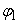and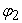.

Location of the endpoint L of member 2 in the coordinate system O, x, y is given by :(1)(2)

The arm is to start from rest at a known position and move to a desired position. The task is to investigate the necessary movement in kinematics pairs which would ensure the relocation of the end point L of member 2 from position L0 (4.5, 0) to position L1 (0, 3) (Figure 1b). At the beginning and end of the movement the velocity and acceleration of point L should be zero.

We try to find the time diagram of angular rotationsandin the form of a fifth degree polynomials :(3)(4)Download asVeiw figureFigures index
Figure 1. Model of manipulator: a) links are connected by two joints; b) starting and final position of two link of manipulator

where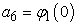and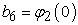are the initial condition at timeand coefficient vectors:(5)(6)

are to be determined to provide the desired motion. The choice of the degree of the polynomials will be explained as the equations of motion are described. For desired initial coordinatesat time t=0 and desired final coordinates at time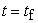,the required values for angles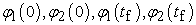can be found using trigonometry. For given values of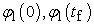andand for given values ofand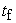, matrix equations are to be set up and solved for coefficient vector a and coefficient vector b. These results are to be used to plot the path of the hand.

The remaining constraints in the arm motion are that the velocity and acceleration of the links are to be zero at the known starting location and desired final location. This implies that the angular velocity and angular acceleration of the two angles are to be zero at timeand. The angular velocity of the first link at time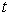is the derivative of the angle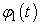with respect to time :(7)

The velocity at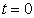is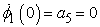(8)

and thus, coefficient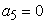. The angular acceleration is the second derivative of the anglewith respect to time: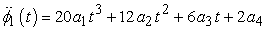(9)

The acceleration atis: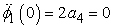(10)

and thus, coefficient. Writing the three constraints onand its derivatives at time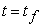in matrix form: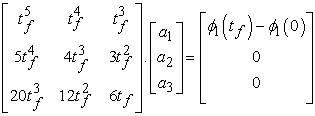(11)

Similarly, for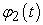: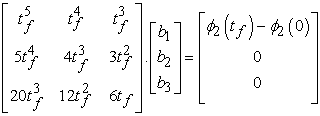(12)

Note that there are three equations and three unknowns for each angle and its two derivatives. This is the reason for choosing a fifth-degree polynomial to control the motion. The lower degree three terms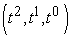have coefficients with value zero to meet the constraints at t=0. This leaves the higher degree three termsand corresponding three unknown coefficients. Adding additional higher degree terms would require additional constraint equations to be able to compute the values of the corresponding coefficients .

Assuming the following initial and final values and link lengths: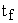=2 s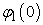=-36,87°= 90°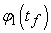= 42,7785°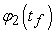= 125,3593°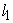=0,36 m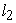=0,27 m

which correspond to a starting hand location of (4.5, 0) and a destination location of (0,3), and plot the path of the end point L of the member 2 .Download asVeiw figureFigures index
Figure 2. Window Figure 1 from Matlab simulation

Time diagram of the trajectory of the point L is shown in Figure 2 and Figure 3 from Matlab solution :Download asVeiw figureFigures index
Figure 3. Calculated path of the point L of the robot hand

Time diagram of angular rotation, angular velocity and angular acceleration of the members is shown in Figure 4 .Download asVeiw figureFigures index
Figure 4. Time diagrams of the mechanical system with kinematical parameters

The constants in the polynomials are determined from the initial and end conditions of the endpoint L. The degree of these polynomials is chosen so that they are able to express all the desired conditions. After substituting the initial and final conditions we obtain the following values of their respective constants: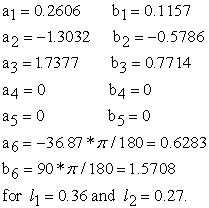### 3. Inverse Problem

While solving the inverse problem we determine the moments M1 and M2 required to obtain the prescribed movement of the mechanical system . Inputs are the prescribed movements of its individual members. Member 2 is to be moved from position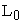to the desired position L1 along trajectory determined in the preceding paragraph. Block diagram of the mechanical system for solving the inverse problem  is in Figure 5.Download asVeiw figureFigures index
Figure 5. Block diagram of the mechanical system in SimMechanics (inverse dynamics)

The block of the Body1 (Figure 6) in SimMechanics scheme is shown :Download asVeiw figureFigures index
Figure 6. Block Parameters: Body1 of the mechanical system in SimMechanics (inverse dynamics)

The block of the Body2 (Figure 7) in SimMechanics scheme:Download asVeiw figureFigures index
Figure 7. Block Parameters: Body2 of the mechanical system in SimMechanics (inverse dynamics)

Time diagrams of torques  in the kinematics pairs M1 and M2 obtained by solving the model in the above block diagram are shown in Figure 8.Download asVeiw figureFigures index
Figure 8. Time diagram of actuator forces of the mechanical systemDownload asVeiw figureFigures index
Figure 9. Time diagram of actuator forces of the mechanical system

### 4. Direct Problem

Direct problem investigates the movement of the mechanical system under the load of the forces obtained in the previous paragraph. Application of the results from the inverse problem on the model in Figure 10 should result in motion as we prescribed it at the beginning of the analysis .Download asVeiw figureFigures index
Figure 10. Block diagram of the mechanical system in SimMechanics (forward dynamics)

The block Joint Initial Condition forin SimMechanics scheme:Download asVeiw figureFigures index
Figure 11. Block Joint Initial Condition in SimMechanics (forward dynamics)

The block Joint Initial Condition forin SimMechanics scheme :Download asVeiw figureFigures index
Figure 12. Block Joint Initial Condition in SimMechanics (forward dynamics)Download asVeiw figureFigures index
Figure 13. Time diagram of SimMechanics simulation

Results obtained from the simulation in Figure 13 correspond with the prescribed motion we determined from the desired location of the endpoint L that are in Figure 4.

### 5. Conclusion

In the work is shown a procedure for solving direct and inverse dynamic problem of the mechanism of double pendulum using modeling in SimMechanics. SimMechanics allows to simulate such mechanical systems which is a common problem in engineering practice. Results are obtained in form of time diagram of the desired variables. Tasks are solved numerically, model is compiled by using block diagrams. Mastering this methodology provides a suitable tool for solving problems of teaching and practice.

### Acknowledgement

This work was supported by grant projects VEGA No. 1/1205/12 and grant projects VEGA No. 1/0937/12.

### References

  The MathWorks,Inc. SimMechanics User’s Guide, November 2002. http://www.mathworks.com.In article  http://www.pwsztar.edu.pl/~r_wiatr/matlab/%28eBook%29%20%20Engineering%20-%20Matlab%20Programming.pdfIn article  Gmiterko, A., 2004. Mechatronics, Emilenia, .In article  Gmiterko, A., Šarga, P., Hroncová, D., 2012. Mechatronika I, Technická univerzita Košice, .In article  Hroncová, D., 2012. Studies of compatible software tools for abstract and concrete design of mechatronic systems and linking them for the purpose of logical and physical modeling, Dissertation thesis, .In article  Karban, P., 2006. Výpočty a simulace v programech Matlab a Simulink. Computer Press, , Česko.In article  Schlotter, M., 2003. Multibody System Simulation with SimMechanics. .In article  Virgala, , Frankovský, P., Kenderová, M.: Friction Effect Analysis of a DC Motor. In: American Journal of Mechanical Engineering. 2013. Vol. 1, no. 1 (2013), p. 1-5In article View Article  Vittek, J., 1997. Matlab pre elektrické pohony, Nakladateľstvo Žilinskej univerzity, Žilina, Slovensko, p. 35-36.In article